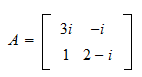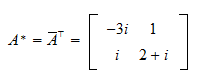StatLect

# Conjugate transpose

It often happens in matrix algebra that we need to both transpose and take the complex conjugate of a matrix. The result of the sequential application of these two operations is called conjugate transpose (or Hermitian transpose). Special symbols are used in the mathematics literature to denote this double operation.## Definition

The conjugate transpose of a matrixis the matrixdefined bywheredenotes transposition and the over-line denotes complex conjugation.

Remember that the complex conjugate of a matrix is obtained by taking the complex conjugate of each of its entries (see the lecture on complex matrices).

In the definition we have used the fact that the order in which transposition and conjugation are performed is irrelevant: whether the sign of the imaginary part of an entry ofis switched before or after moving the entry to a different position does not change the final result.

Example Define the matrixIts conjugate isand its conjugate transpose is## Symbols

Several different symbols are used in the literature as alternatives to thesymbol we have used thus far.

The most common alternatives are thesymbol (for Hermitian):and the dagger:## Properties

The properties of conjugate transposition are immediate consequences of the properties of transposition and conjugation. We therefore list some of them without proofs.

For any two matricesandsuch that the operations below are well-defined and any scalar, we have that

•••••providedis a square invertible matrix

## Solved exercises

Below you can find some exercises with explained solutions.

### Exercise 1

Let the vectorbe defined byCompute the productSolution

The conjugate transpose ofisand the product is### Exercise 2

Let the matrixbe defined byCompute its conjugate transpose.

Solution

We have thatThe book

Most of the learning materials found on this website are now available in a traditional textbook format.

Glossary entries
Share